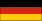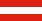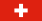k = sequence
 m = n =
 x = k(n2-m2) y = 2kmn z = k(n2+m2)Pythagorean Triples

A pythagorean triplet ("PT") consists of three natural numbers x, y and z with x2 + y2 = z2. PT's with greatest common divisor 1 ("PPT's") are of particular interest.
Theorem 1 Every PT can be obtained in a unique way as a product of a PPT and a natural number k.
Theorem 2 In every PPT ( x | y | z ) one of the numbers x or y ("legs") is even and the other one is odd. (Let x always be the odd leg.)
Theorem 3 For every PPT ( x | y | z ) there exists one and only one pair ( m | n ) of relatively prime natural numbers of different parity (ie.: one of the numbers is even and the other is odd) with m < n, such that:
x = n2 - m2, y = 2mn , z = n2 + m2   resp.  1/2(z - x) = m2, 1/2(z + x) = n2.

Theorem 4 Every PPT can in a unique way be obtained from ( 3 | 4 | 5 ) by application of a "sequence" of transformations A, B or C defined by:

A: (x|y|z) --> ( x-2y+2z| 2x-y+2z| 2x-2y+3z)   [or (m|n) --> (n|2n-m)]
B: (x|y|z) --> ( x+2y+2z| 2x+y+2z| 2x+2y+3z)   [or (m|n) --> (n|2n+m)]
C: (x|y|z) --> (-x+2y+2z|-2x+y+2z|-2x+2y+3z)   [or (m|n) --> (m|2m+n)]

 to other interesting mathematical topics ... for more information about the pairs ( m | n ) and the transformations A, B, C see: number theory interactive .. zur Seite in deutscher Sprache:Explanations for the "PT-calculator" (Java Script required)
k =: the greatest common divisor k of a PT is edited here.
sequence: one can choose any squence of letters A, B or C; a click at the button "take sequence" causes suczessive application of the choosen transformations (beginning from the right side) to (3|4|5) and multiplication with k. The resulting PT, and the pair (m|n) will be displayed.
m =    n = : input for m and n: button: "take m and n". The PT and the corresponding sequence will be shown.
x =  y =  z = : the x-y-windows are input/output windows (button: "take x and y"); output: k, the sequence, z, and (m|n).
Buttons: A B C : edition of a sequence by mouseclick.
Button: Reduce : to reduce means: the last (left) transformation of a sequence will be reversed. Reduction ist a one to one application: for every PT there exists exactly one reduced PT. Finally division by k is done. Suczessive reduction always ends with (3|4|5) (Theorem 5).
Button: Reset : the original state will be restored.